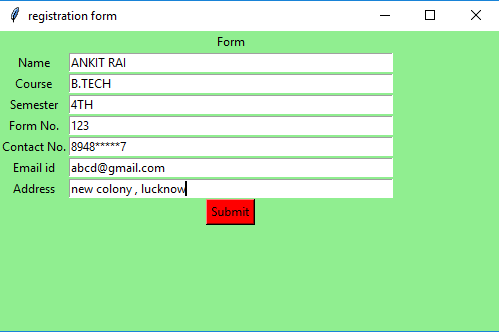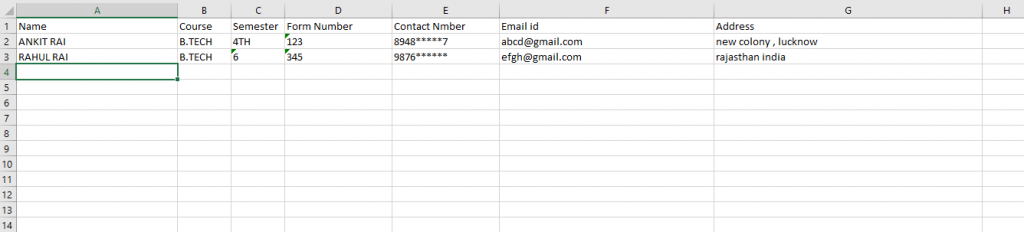# Simple registration form using Python Tkinter

• Difficulty Level : Medium
• Last Updated : 09 Sep, 2021

Prerequisites: Tkinter Introduction, openpyxl module.
Python provides the Tkinter toolkit to develop GUI applications. Now, it’s upto the imagination or necessity of developer, what he/she want to develop using this toolkit. Let’s make a simple information form GUI application using Tkinter. In this application, User has to fill up the required information, and that information is automatically written into an excel file.

Firstly, create an empty excel file, after that pass an absolute path of the excel file in the program so that the program is able to access that excel file.

Below is the implementation :

## Python3

 `# import openpyxl and tkinter modules``from` `openpyxl ``import` `*``from` `tkinter ``import` `*` `# globally declare wb and sheet variable` `# opening the existing excel file``wb ``=` `load_workbook(``'C:\\Users\\Admin\\Desktop\\excel.xlsx'``)` `# create the sheet object``sheet ``=` `wb.active`  `def` `excel():``    ` `    ``# resize the width of columns in``    ``# excel spreadsheet``    ``sheet.column_dimensions[``'A'``].width ``=` `30``    ``sheet.column_dimensions[``'B'``].width ``=` `10``    ``sheet.column_dimensions[``'C'``].width ``=` `10``    ``sheet.column_dimensions[``'D'``].width ``=` `20``    ``sheet.column_dimensions[``'E'``].width ``=` `20``    ``sheet.column_dimensions[``'F'``].width ``=` `40``    ``sheet.column_dimensions[``'G'``].width ``=` `50` `    ``# write given data to an excel spreadsheet``    ``# at particular location``    ``sheet.cell(row``=``1``, column``=``1``).value ``=` `"Name"``    ``sheet.cell(row``=``1``, column``=``2``).value ``=` `"Course"``    ``sheet.cell(row``=``1``, column``=``3``).value ``=` `"Semester"``    ``sheet.cell(row``=``1``, column``=``4``).value ``=` `"Form Number"``    ``sheet.cell(row``=``1``, column``=``5``).value ``=` `"Contact Number"``    ``sheet.cell(row``=``1``, column``=``6``).value ``=` `"Email id"``    ``sheet.cell(row``=``1``, column``=``7``).value ``=` `"Address"`  `# Function to set focus (cursor)``def` `focus1(event):``    ``# set focus on the course_field box``    ``course_field.focus_set()`  `# Function to set focus``def` `focus2(event):``    ``# set focus on the sem_field box``    ``sem_field.focus_set()`  `# Function to set focus``def` `focus3(event):``    ``# set focus on the form_no_field box``    ``form_no_field.focus_set()`  `# Function to set focus``def` `focus4(event):``    ``# set focus on the contact_no_field box``    ``contact_no_field.focus_set()`  `# Function to set focus``def` `focus5(event):``    ``# set focus on the email_id_field box``    ``email_id_field.focus_set()`  `# Function to set focus``def` `focus6(event):``    ``# set focus on the address_field box``    ``address_field.focus_set()`  `# Function for clearing the``# contents of text entry boxes``def` `clear():``    ` `    ``# clear the content of text entry box``    ``name_field.delete(``0``, END)``    ``course_field.delete(``0``, END)``    ``sem_field.delete(``0``, END)``    ``form_no_field.delete(``0``, END)``    ``contact_no_field.delete(``0``, END)``    ``email_id_field.delete(``0``, END)``    ``address_field.delete(``0``, END)`  `# Function to take data from GUI``# window and write to an excel file``def` `insert():``    ` `    ``# if user not fill any entry``    ``# then print "empty input"``    ``if` `(name_field.get() ``=``=` `"" ``and``        ``course_field.get() ``=``=` `"" ``and``        ``sem_field.get() ``=``=` `"" ``and``        ``form_no_field.get() ``=``=` `"" ``and``        ``contact_no_field.get() ``=``=` `"" ``and``        ``email_id_field.get() ``=``=` `"" ``and``        ``address_field.get() ``=``=` `""):``            ` `        ``print``(``"empty input"``)` `    ``else``:` `        ``# assigning the max row and max column``        ``# value upto which data is written``        ``# in an excel sheet to the variable``        ``current_row ``=` `sheet.max_row``        ``current_column ``=` `sheet.max_column` `        ``# get method returns current text``        ``# as string which we write into``        ``# excel spreadsheet at particular location``        ``sheet.cell(row``=``current_row ``+` `1``, column``=``1``).value ``=` `name_field.get()``        ``sheet.cell(row``=``current_row ``+` `1``, column``=``2``).value ``=` `course_field.get()``        ``sheet.cell(row``=``current_row ``+` `1``, column``=``3``).value ``=` `sem_field.get()``        ``sheet.cell(row``=``current_row ``+` `1``, column``=``4``).value ``=` `form_no_field.get()``        ``sheet.cell(row``=``current_row ``+` `1``, column``=``5``).value ``=` `contact_no_field.get()``        ``sheet.cell(row``=``current_row ``+` `1``, column``=``6``).value ``=` `email_id_field.get()``        ``sheet.cell(row``=``current_row ``+` `1``, column``=``7``).value ``=` `address_field.get()` `        ``# save the file``        ``wb.save(``'C:\\Users\\Admin\\Desktop\\excel.xlsx'``)` `        ``# set focus on the name_field box``        ``name_field.focus_set()` `        ``# call the clear() function``        ``clear()`  `# Driver code``if` `__name__ ``=``=` `"__main__"``:``    ` `    ``# create a GUI window``    ``root ``=` `Tk()` `    ``# set the background colour of GUI window``    ``root.configure(background``=``'light green'``)` `    ``# set the title of GUI window``    ``root.title(``"registration form"``)` `    ``# set the configuration of GUI window``    ``root.geometry(``"500x300"``)` `    ``excel()` `    ``# create a Form label``    ``heading ``=` `Label(root, text``=``"Form"``, bg``=``"light green"``)` `    ``# create a Name label``    ``name ``=` `Label(root, text``=``"Name"``, bg``=``"light green"``)` `    ``# create a Course label``    ``course ``=` `Label(root, text``=``"Course"``, bg``=``"light green"``)` `    ``# create a Semester label``    ``sem ``=` `Label(root, text``=``"Semester"``, bg``=``"light green"``)` `    ``# create a Form No. label``    ``form_no ``=` `Label(root, text``=``"Form No."``, bg``=``"light green"``)` `    ``# create a Contact No. label``    ``contact_no ``=` `Label(root, text``=``"Contact No."``, bg``=``"light green"``)` `    ``# create a Email id label``    ``email_id ``=` `Label(root, text``=``"Email id"``, bg``=``"light green"``)` `    ``# create a address label``    ``address ``=` `Label(root, text``=``"Address"``, bg``=``"light green"``)` `    ``# grid method is used for placing``    ``# the widgets at respective positions``    ``# in table like structure .``    ``heading.grid(row``=``0``, column``=``1``)``    ``name.grid(row``=``1``, column``=``0``)``    ``course.grid(row``=``2``, column``=``0``)``    ``sem.grid(row``=``3``, column``=``0``)``    ``form_no.grid(row``=``4``, column``=``0``)``    ``contact_no.grid(row``=``5``, column``=``0``)``    ``email_id.grid(row``=``6``, column``=``0``)``    ``address.grid(row``=``7``, column``=``0``)` `    ``# create a text entry box``    ``# for typing the information``    ``name_field ``=` `Entry(root)``    ``course_field ``=` `Entry(root)``    ``sem_field ``=` `Entry(root)``    ``form_no_field ``=` `Entry(root)``    ``contact_no_field ``=` `Entry(root)``    ``email_id_field ``=` `Entry(root)``    ``address_field ``=` `Entry(root)` `    ``# bind method of widget is used for``    ``# the binding the function with the events` `    ``# whenever the enter key is pressed``    ``# then call the focus1 function``    ``name_field.bind(``""``, focus1)` `    ``# whenever the enter key is pressed``    ``# then call the focus2 function``    ``course_field.bind(``""``, focus2)` `    ``# whenever the enter key is pressed``    ``# then call the focus3 function``    ``sem_field.bind(``""``, focus3)` `    ``# whenever the enter key is pressed``    ``# then call the focus4 function``    ``form_no_field.bind(``""``, focus4)` `    ``# whenever the enter key is pressed``    ``# then call the focus5 function``    ``contact_no_field.bind(``""``, focus5)` `    ``# whenever the enter key is pressed``    ``# then call the focus6 function``    ``email_id_field.bind(``""``, focus6)` `    ``# grid method is used for placing``    ``# the widgets at respective positions``    ``# in table like structure .``    ``name_field.grid(row``=``1``, column``=``1``, ipadx``=``"100"``)``    ``course_field.grid(row``=``2``, column``=``1``, ipadx``=``"100"``)``    ``sem_field.grid(row``=``3``, column``=``1``, ipadx``=``"100"``)``    ``form_no_field.grid(row``=``4``, column``=``1``, ipadx``=``"100"``)``    ``contact_no_field.grid(row``=``5``, column``=``1``, ipadx``=``"100"``)``    ``email_id_field.grid(row``=``6``, column``=``1``, ipadx``=``"100"``)``    ``address_field.grid(row``=``7``, column``=``1``, ipadx``=``"100"``)` `    ``# call excel function``    ``excel()` `    ``# create a Submit Button and place into the root window``    ``submit ``=` `Button(root, text``=``"Submit"``, fg``=``"Black"``,``                            ``bg``=``"Red"``, command``=``insert)``    ``submit.grid(row``=``8``, column``=``1``)` `    ``# start the GUI``    ``root.mainloop()`

Output :My Personal Notes arrow_drop_up